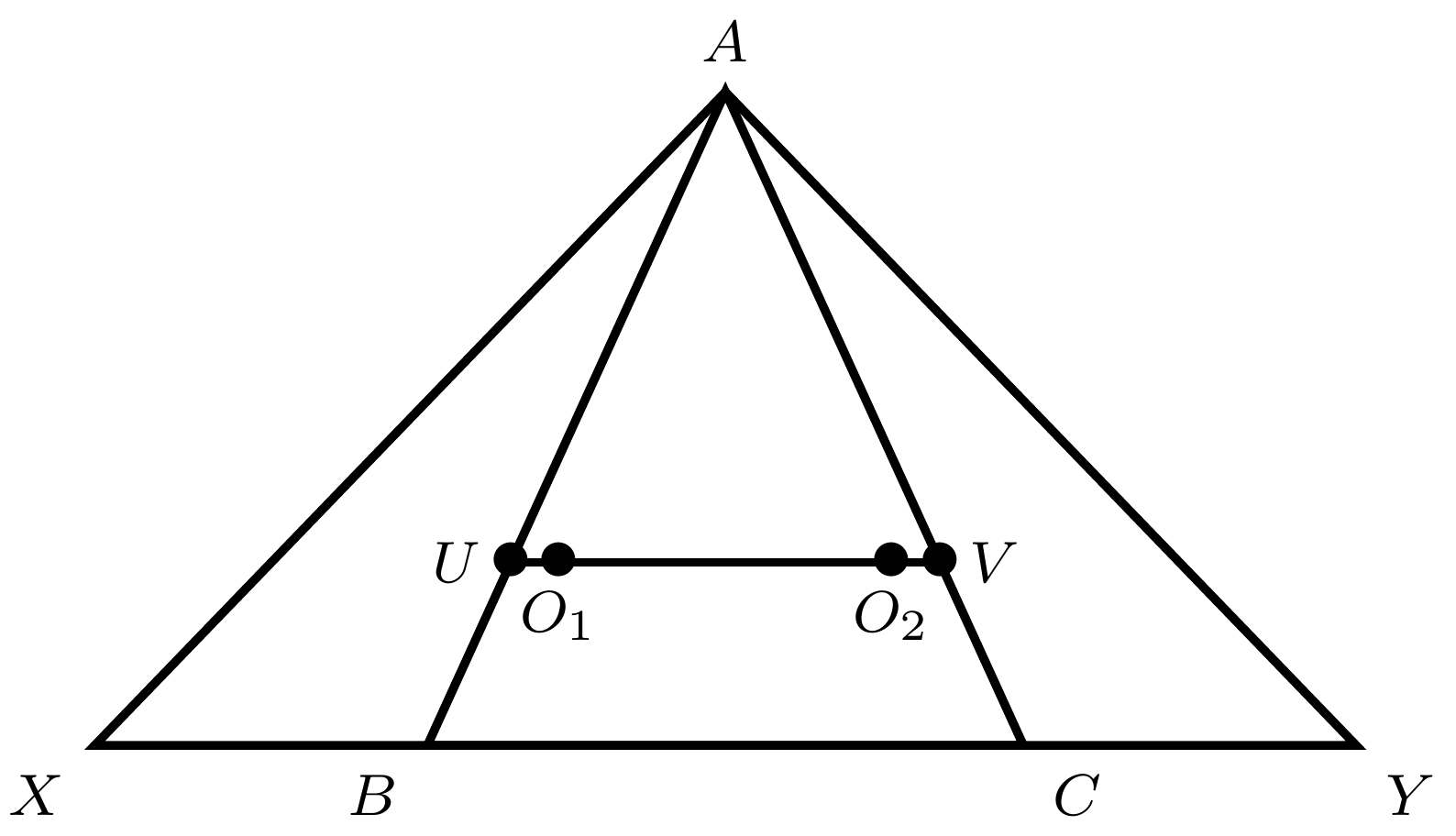###### back to index | new

Compute the value of $$\sum_{n=2019}^\infty\frac{1}{\binom{n}{2019}}$$

Show that $$\binom{n}{1}-\frac{1}{2}\binom{n}{2}+\frac{1}{3}\binom{n}{3}-\cdots+(-1)^{n+1}\binom{n}{n}= 1+\frac{1}{2}+\frac{1}{3}+\cdots+\frac{1}{n}$$

Let $n$ be a positive integer. Show that $$\Big(1+\frac{1}{3}\Big)\Big(1+\frac{1}{3^2}\Big)\cdots\Big(1+\frac{1}{3^n}\Big) < 2$$

Let $\alpha$ and $\beta$ be the two roots of the equation $x^2 -x - 1=0$. If $$a_n = \frac{\alpha^n - \beta^n}{\alpha -\beta}\quad(n=1, 2, \cdots)$$ Show that - For any positive integer $n$, it always hold $a_{n+2}=a_{n+1}+a_n$ - Find all positive integers $a, b$ $( a < b )$ satisfying $b\mid a_n-2na^n$ holds for any positive integer $n$

Let $\{a_n\}$ be an increasing geometric sequence satisfying $a_1+a_2=6$ and $a_3+a_4=24$. Let $\{b_n\}$ be another sequence satisfying $b_n=\frac{a_n}{(a_n-1)^2}$. If $T_n$ is the sum of first $n$ terms in $\{b_n\}$, show that for any positive integer $n$, it always holds that $T_n < 3$.

If a sequence $\{a_n\}$ satisfies $a_1=1$ and $a_{n+1}=\frac{1}{16}\big(1+4a_n+\sqrt{1+24a_n}\big)$, find the general term of $a_n$.

Let sequence $\{a_n\}$ satisfy $a_0=1$ and $a_n=\frac{\sqrt{1+a_{n-1}^2}-1}{a_{n-1}}$. Prove $a_n > \frac{\pi}{2^{n+2}}$.

Let ${{a}_{2}}, {{a}_{3}}, \cdots, {{a}_{n}}$ be positive real numbers that satisfy ${{a}_{2}}\cdot {{a}_{3}}\cdots {{a}_{n}}=1$ . Prove that $$(a_2+1)^2\cdot (a_3+1)^3\cdots (a_n+1)^n\ge n^n$$

Let $a, b, c$ be the lengths of the sides of triangle $ABC$. Show that $$\sqrt{a}(c+a-b) + \sqrt{b}(a+b-c)+\sqrt{c}(b+c-a)\le\sqrt{(a^2 + b^2 + c^2)(a+b+c)}$$

Let $x, y, z$ be three distinct positive real numbers such that $x+\sqrt{y+\sqrt{z}}=z+\sqrt{y+\sqrt{x}}$. Show that $40xz<1$

(Rearrangement Theorem) Let $a_1, a_2, \cdots, a_n$ and $b_1, b_2, \cdots, b_n$ be sequences of positive real numbers, and let $c_1, c_2, \cdots, c_n$ be a permutation of $b_1, b_2, \cdots, b_n$. The sum $S=a_1b_1+a_2b_2+\cdots+a_nb_n$ is maximal if the two sequences $a_1, a_2, \cdots, a_n$ and $b_1, b_2, \cdots, b_n$ are sorted in the same way and minimal if the two sequences are sorted oppositely, one increasing and the other decreasing.

The number $21982145917308330487013369$ is the thirteenth power of a positive integer. Which positive integer?

Compute $$\sqrt{1+2\sqrt{1+3\sqrt{1+4\sqrt{1+\cdots}}}}$$

Simplify $\sqrt{5\sqrt{3}+6\sqrt{2}}$.

Simplify $\sqrt{\sqrt{5}-\sqrt{4}}$.

Simplify $\sqrt{6+\sqrt{81}+\sqrt{9}}$.

Find all $x$ such that $\displaystyle\sum_{k=1}^{\infty}kx^k=20$.

Simplify $$\sin{x} + \sin{2x} + \cdots +\sin{nx}$$ and $$\cos{x} + \cos{2x} + \cdots + \cos{nx}$$

Solve the equation $\cos\theta + \cos 2\theta + \cos 3\theta = \sin \theta +\sin 2\theta + \sin 3\theta$.

Let $S_n$ be the minimal value of $\displaystyle\sum_{k=1}^n\sqrt{a_k^2+b_k^2}$ where $\{a_k\}$ is an arithmetic sequence whose first term is $4$ and common difference is $8$. $b_1, b_2,\cdots, b_n$ are positive real numbers satisfying $\displaystyle\sum_{k=1}^nb_k=17$. If there exist a positive integer $n$ such that $S_n$ is also an integer, find $n$.

Solve the equation $\cos^2 x + \cos^2 2x +\cos^2 3x=1$ in $(0, 2\pi)$.

Prove for every positive integer $n$ and real number $x\ne \frac{k\pi}{2^t}$ where $t =0, 1, 2,\cdots$ and $k$ is an integer, the following relation always holds: $$\frac{1}{\sin 2x}+\frac{1}{\sin 4x} + \cdots +\frac{1}{\sin 2^nx}=\frac{1}{\tan x}-\frac{1}{\tan 2^nx}$$

Without using a calculator, find the value of $\cos\frac{\pi}{13}+\cos\frac{3\pi}{13}+\cos\frac{9\pi}{13}$.

As shown, points $X$ and $Y$ are on the extension of $BC$ in $\triangle{ABC}$ such that the order of these four points are $X$, $B$, $C$, and $Y$. Meanwhile, they satisfy the relation $BX\cdot AC = CY\cdot AB$. Let $O_1$ and $O_2$ be the circumcenters of $\triangle{ACX}$ and $\triangle{ABY}$, respectively. If $O_1O_2$ intersects $AB$ and $AC$ at $U$ and $V$, respectively, show that $\triangle{AUV}$ is isosceles.Let real numbers $a_1$, $a_2$, $\cdots$, $a_{2016}$ satisfy $9a_i\ge 11a_{i+1}^2$ for $i=1, 2,\cdots, 2015$. Define $a_{2017}=a_1$, find the maximum value of $$P=\displaystyle\prod_{i=1}^{2016}(a_i-a_{i+1}^2)$$